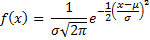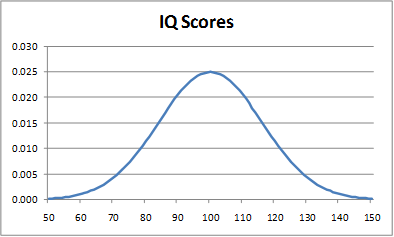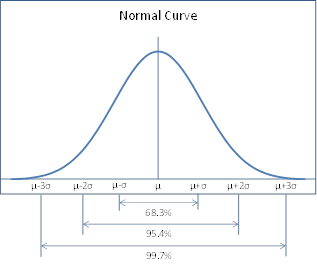# Basic Characteristics of the Normal Distribution

Definition 1: The probability density function of the normal distribution is defined as:Here is the constant e = 2.7183…, and is the constant π = 3.1415… .

The normal distribution is completely determined by the parameters µ and σ. It turns out that µ is the mean of the normal distribution and σ is the standard deviation. We use the abbreviation N(µ, σ) to refer to a normal distribution with mean µ and standard deviation σ.

As we shall see, the normal distribution occurs frequently and is very useful in statistics.

Excel Functions: Excel provides the following functions regarding the normal distribution:

NORMDIST(x, μ, σ, cum) where cum takes the value TRUE or FALSE

NORMDIST(x, μ, σ, FALSE) = probability density function value f(x) for the normal distribution

NORMDIST(x, μ, σ, TRUE) = cumulative probability distribution value F(x) for the normal distribution

NORMINV(p, μ, σ) is the inverse of NORMDIST(x, μ, σ, TRUE)

NORMINV(p, μ, σ) = the value x such that NORMDIST(x, μ, σ, TRUE) = p

Excel 2010/2013/2016 provide the following additional functions: NORM.DIST, which is equivalent to NORMDIST, and NORM.INV, which is equivalent to NORMINV.

Example 1: Create a graph of the distribution of IQ scores using the Stanford-Binet scale.

This distribution is known to be the normal distribution N(100, 16). To create the graph, we first create a table with the values of the probability density function f(x) for for values of x = 50, 51, …, 150. This table begins as shown in Figure 1.Figure 1 – Probability density function for IQ

The value of f(x) for each x is calculated using the NORMDIST function with cum = FALSE. The probability density curve is created as a line chart using the techniques described in Line Charts. From Figure 2, you can see that the curve in this chart has the characteristic bell shape of the normal distribution.Figure 2 – IQ scores as normal curve

Observation: As can be seen from Figure 2, the area under the curve to the right of 100 is equal to the area under the curve to left of 100; this makes 100 the mean. Since the normal curve is symmetric about the mean, it follows that the median is also 100. Since the curve reaches its highest point at 100, it follows that the mode is also 100.

Observation: The basic parameters of the normal distribution are as follows:

• Mean = median = mode = µ
• Standard deviation = σ
• Skewness = kurtosis = 0

The function is symmetric about the mean with inflection points (i.e. the points where there curve changes from concave up to concave down or from concave down to concave up) at x = μ ± σ.

As can be seen from Figure 3, the area under the curve in the interval μ – σ < x < μ + σ is approximately 68.26% of the total area under the curve. The area under the curve in the interval μ – 2σ < x < μ + 2σ is approximately 95.44% of the total area under the curve and the area under the curve in the interval μ – 3σ < x < μ + 3σ is approximately 99.74% of the area under the curve.Figure 3 – Areas under normal curve

Given the symmetry of the curve, this means that the area under the curve where x > μ + σ is 15.87%, i.e. (100% – 68.26%) / 2. The area under the curve where x > μ + 2σ is 2.28% and the area under the curve where x > μ + 3σ is 0.13%.

It also turns out that 95% of the area under the curve is in the interval -1.96 < x < 1.96. This will be important when considering the critical value for α = .05.

Property 1: If x has normal distribution N(μ, σ) then the linear transform y = ax + b, where a and  b are constants, has normal distribution N(aμ+b, aσ).

Property 2: If x1 and x2 are independent random variables, and x1 has normal distribution N(μ1, σ1)and x2 has normal distribution N(μ2, σ2) then x1 + x2 has normal distribution N(μ1+μ2σ) whereExample 2: A charity group prepares sandwiches for the poor. The weights of the sandwiches are distributed normally with mean 150 grams and standard deviation of 25 grams. One sandwich is chosen at random (this is a random sample of size one). What is the probability that this sandwich will weigh between 145 and 155 grams?

NORMDIST(145, 150, 25, TRUE) = .42074 = probability that weight is less than 145 grams

NORMDIST(155, 150, 25, TRUE) = .57926 = probability that weight is less than 155 grams

The answer therefore = .57926 – . 42074 = .15852 = 15.85%.

Statistics with R for Business Analysts – Normal Distribution

# Personal Career & Learning Guide for Data Analyst, Data Engineer and Data Scientist

## Applied Machine Learning & Data Science Projects and Coding Recipes for Beginners

A list of FREE programming examples together with eTutorials & eBooks @ SETScholars

# Projects and Coding Recipes, eTutorials and eBooks: The best All-in-One resources for Data Analyst, Data Scientist, Machine Learning Engineer and Software Developer

Topics included: Classification, Clustering, Regression, Forecasting, Algorithms, Data Structures, Data Analytics & Data Science, Deep Learning, Machine Learning, Programming Languages and Software Tools & Packages.
(Discount is valid for limited time only)`Disclaimer: The information and code presented within this recipe/tutorial is only for educational and coaching purposes for beginners and developers. Anyone can practice and apply the recipe/tutorial presented here, but the reader is taking full responsibility for his/her actions. The author (content curator) of this recipe (code / program) has made every effort to ensure the accuracy of the information was correct at time of publication. The author (content curator) does not assume and hereby disclaims any liability to any party for any loss, damage, or disruption caused by errors or omissions, whether such errors or omissions result from accident, negligence, or any other cause. The information presented here could also be found in public knowledge domains.`

# Learn by Coding: v-Tutorials on Applied Machine Learning and Data Science for Beginners

Please do not waste your valuable time by watching videos, rather use end-to-end (Python and R) recipes from Professional Data Scientists to practice coding, and land the most demandable jobs in the fields of Predictive analytics & AI (Machine Learning and Data Science).

The objective is to guide the developers & analysts to “Learn how to Code” for Applied AI using end-to-end coding solutions, and unlock the world of opportunities!